Search by Topic

Resources tagged with Working systematically similar to Domino Number Patterns:

Filter by: Content type:
Age range:
Challenge level:

There are 321 results

Broad Topics > Using, Applying and Reasoning about Mathematics > Working systematicallyOne of Thirty-six

Age 5 to 7 Challenge Level:

Can you find the chosen number from the grid using the clues?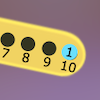Are You Well Balanced?

Age 5 to 7 Challenge Level:

Can you work out how to balance this equaliser? You can put more than one weight on a hook.1 to 8

Age 7 to 11 Challenge Level:

Place the numbers 1 to 8 in the circles so that no consecutive numbers are joined by a line.Number Detective

Age 5 to 11 Challenge Level:

Follow the clues to find the mystery number.Arrangements

Age 7 to 11 Challenge Level:

Is it possible to place 2 counters on the 3 by 3 grid so that there is an even number of counters in every row and every column? How about if you have 3 counters or 4 counters or....?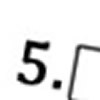Greater Than or Less Than?

Age 7 to 11 Challenge Level:

Use the numbers and symbols to make this number sentence correct. How many different ways can you find?Birthday Cake Candles

Age 7 to 11 Challenge Level:

This challenge involves calculating the number of candles needed on birthday cakes. It is an opportunity to explore numbers and discover new things.Seven Flipped

Age 7 to 11 Challenge Level:

Investigate the smallest number of moves it takes to turn these mats upside-down if you can only turn exactly three at a time.Being Resourceful - Primary Number

Age 5 to 11 Challenge Level:

Number problems at primary level that require careful consideration.ABC

Age 7 to 11 Challenge Level:

In the multiplication calculation, some of the digits have been replaced by letters and others by asterisks. Can you reconstruct the original multiplication?Trebling

Age 7 to 11 Challenge Level:

Can you replace the letters with numbers? Is there only one solution in each case?Curious Number

Age 7 to 11 Challenge Level:

Can you order the digits from 1-3 to make a number which is divisible by 3 so when the last digit is removed it becomes a 2-figure number divisible by 2, and so on?Growing Garlic

Age 5 to 7 Challenge Level:

Ben and his mum are planting garlic. Use the interactivity to help you find out how many cloves of garlic they might have had.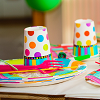Sitting Round the Party Tables

Age 5 to 11 Challenge Level:

Sweets are given out to party-goers in a particular way. Investigate the total number of sweets received by people sitting in different positions.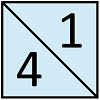Jumping Squares

Age 5 to 7 Challenge Level:

In this problem it is not the squares that jump, you do the jumping! The idea is to go round the track in as few jumps as possible.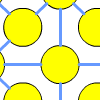One to Fifteen

Age 7 to 11 Challenge Level:

Can you put the numbers from 1 to 15 on the circles so that no consecutive numbers lie anywhere along a continuous straight line?Lots of Lollies

Age 5 to 7 Challenge Level:

Frances and Rishi were given a bag of lollies. They shared them out evenly and had one left over. How many lollies could there have been in the bag?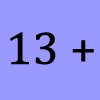What Could it Be?

Age 5 to 7 Challenge Level:

In this calculation, the box represents a missing digit. What could the digit be? What would the solution be in each case?All the Digits

Age 7 to 11 Challenge Level:

This multiplication uses each of the digits 0 - 9 once and once only. Using the information given, can you replace the stars in the calculation with figures?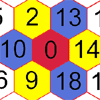Nineteen Hexagons

Age 5 to 7 Challenge Level:

In this maze of hexagons, you start in the centre at 0. The next hexagon must be a multiple of 2 and the next a multiple of 5. What are the possible paths you could take?Page Numbers

Age 7 to 11 Short Challenge Level:

Exactly 195 digits have been used to number the pages in a book. How many pages does the book have?Multiply Multiples 3

Age 7 to 11 Challenge Level:

Have a go at balancing this equation. Can you find different ways of doing it?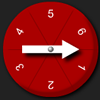Two Spinners

Age 5 to 7 Challenge Level:

What two-digit numbers can you make with these two dice? What can't you make?Multiply Multiples 1

Age 7 to 11 Challenge Level:

Can you complete this calculation by filling in the missing numbers? In how many different ways can you do it?Multiply Multiples 2

Age 7 to 11 Challenge Level:

Can you work out some different ways to balance this equation?Six Is the Sum

Age 7 to 11 Challenge Level:

What do the digits in the number fifteen add up to? How many other numbers have digits with the same total but no zeros?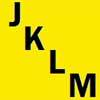Plate Spotting

Age 7 to 11 Challenge Level:

I was in my car when I noticed a line of four cars on the lane next to me with number plates starting and ending with J, K, L and M. What order were they in?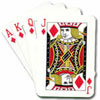Counting Cards

Age 7 to 11 Challenge Level:

A magician took a suit of thirteen cards and held them in his hand face down. Every card he revealed had the same value as the one he had just finished spelling. How did this work?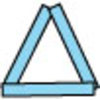Sticks and Triangles

Age 7 to 11 Challenge Level:

Using different numbers of sticks, how many different triangles are you able to make? Can you make any rules about the numbers of sticks that make the most triangles?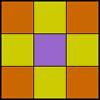Symmetry Challenge

Age 7 to 11 Challenge Level:

Systematically explore the range of symmetric designs that can be created by shading parts of the motif below. Use normal square lattice paper to record your results.Tri.'s

Age 7 to 11 Challenge Level:

How many triangles can you make on the 3 by 3 pegboard?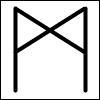Ancient Runes

Age 7 to 11 Challenge Level:

The Vikings communicated in writing by making simple scratches on wood or stones called runes. Can you work out how their code works using the table of the alphabet?Age 7 to 11 Challenge Level:

Can you put plus signs in so this is true? 1 2 3 4 5 6 7 8 9 = 99 How many ways can you do it?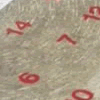All Seated

Age 7 to 11 Challenge Level:

Look carefully at the numbers. What do you notice? Can you make another square using the numbers 1 to 16, that displays the same properties?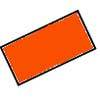Two by One

Age 7 to 11 Challenge Level:

An activity making various patterns with 2 x 1 rectangular tiles.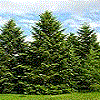Family Tree

Age 7 to 11 Challenge Level:

Use the clues to find out who's who in the family, to fill in the family tree and to find out which of the family members are mathematicians and which are not.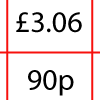How Much Did it Cost?

Age 7 to 11 Challenge Level:

Use your logical-thinking skills to deduce how much Dan's crisps and ice-cream cost altogether.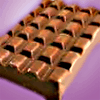Chocoholics

Age 7 to 11 Challenge Level:

George and Jim want to buy a chocolate bar. George needs 2p more and Jim need 50p more to buy it. How much is the chocolate bar?Uncanny Triangles

Age 7 to 11 Challenge Level:

Can you help the children find the two triangles which have the lengths of two sides numerically equal to their areas?Here to There 1 2 3

Age 5 to 7 Challenge Level:

Move from the START to the FINISH by moving across or down to the next square. Can you find a route to make these totals?Zargon Glasses

Age 7 to 11 Challenge Level:

Zumf makes spectacles for the residents of the planet Zargon, who have either 3 eyes or 4 eyes. How many lenses will Zumf need to make all the different orders for 9 families?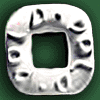Polo Square

Age 7 to 11 Challenge Level:

Arrange eight of the numbers between 1 and 9 in the Polo Square below so that each side adds to the same total.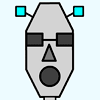Robot Monsters

Age 5 to 7 Challenge Level:

Use these head, body and leg pieces to make Robot Monsters which are different heights.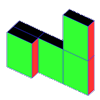Cereal Packets

Age 7 to 11 Challenge Level:

How can you put five cereal packets together to make different shapes if you must put them face-to-face?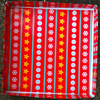Cover the Tray

Age 7 to 11 Challenge Level:

These practical challenges are all about making a 'tray' and covering it with paper.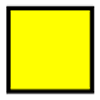Shaping Up

Age 7 to 11 Challenge Level:

Are all the possible combinations of two shapes included in this set of 27 cards? How do you know?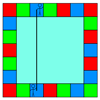Ribbon Squares

Age 7 to 11 Challenge Level:

What is the largest 'ribbon square' you can make? And the smallest? How many different squares can you make altogether?Seven Pots of Plants

Age 7 to 11 Challenge Level:

There are seven pots of plants in a greenhouse. They have lost their labels. Perhaps you can help re-label them.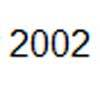Palindromic Date

Age 7 to 11 Challenge Level:

What is the date in February 2002 where the 8 digits are palindromic if the date is written in the British way?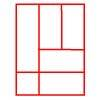Is a Square a Rectangle?

Age 7 to 11 Challenge Level:

How many rectangles can you find in this shape? Which ones are differently sized and which are 'similar'?